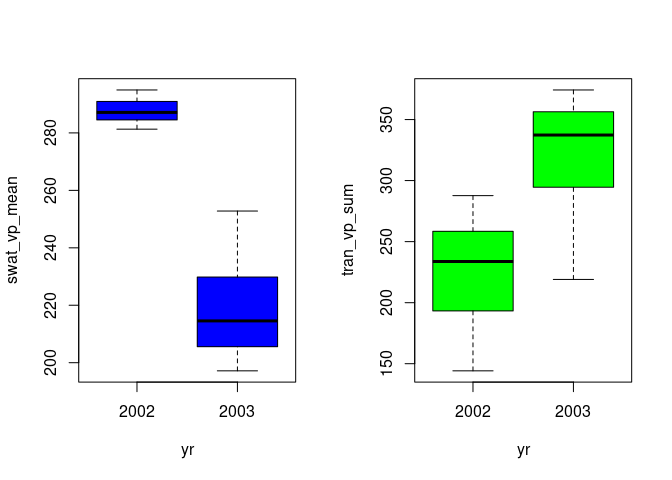# Multi-run simulations in LWFBrook90R

## Introduction

With ‘LWFBrook90R’, parallelized multi-run simulations can be performed conveniently, extending the basic single-run applications using the function run_LWFB90() described in the introductory vignette. Two different multi-run functions exist for two different problems:

1. Perform Monte-Carlo simulations with single parameters set up for variation, and
2. simulations over multiple locations, parameter sets, or climate scenarios.

For the first case, the function run_multi_LWFB90() is available. The second problem can be tackled using the function run_multisite_LWFB90(), which is described in detail in the vignette ‘Multi-Site simulations’.

Both functions are wrapper functions for run_LWFB90() and allow for parallel processing of tasks, using a specified number of CPUs to speed up the execution of a multi-run simulation. They return lists containing the individual single run simulation results, as they are returned by run_LWFB90(). These result-lists can become very large if many simulations are performed, and the selected output comprises daily data sets and especially the individual soil layers’ daily soil moisture states. Huge amounts of data produced can overload the memory, this vignette therefore starts with a data management section on how to make best use of the output_fun-argument of run_LWFB90() to reduce the amount of data returned.

library(LWFBrook90R)
library(data.table)

## Data management (i): output_fun-argument

To minimize memory allocation, it is recommended to reduce the selected output to a minimum and make use of the output_fun-argument of run_LWFB90(). With this argument, it is possible to pass custom functions to run_LWFB90(), which directly perform on the simulation output list object. With rtrn_output = FALSE, the original simulation output (output, layer_output) then can be discarded, and only the results from the output_fun-argument are returned. This can be very useful for model calibration or sensitivity analyses tasks comprising ten thousands of simulations in a Monte-Carlo setting. With this magnitude, memory allocation is critical, and only a relatively small output can be returned for each individual simulation (e.g., a measure of agreement between simulated and observed values). Similarly, it is possible to define functions for custom output aggregation, or to redirect the simulation output to a file or database, as we will see later.

To demonstrate the usage of the output_fun-argument, we perform a Monte-Carlo simulation using the function run_multi_LWFB90(), and define a function that returns annual mean soil water storage and transpiration during the growing season. In a first step, the function integrates depth-specific soil moisture to soil water storage down to specified soil layer (tolayer, passed via ... to output_fun), and in a second step calculates mean soil water storage over the growing season, along with the sum of transpiration. The growing season thereby is defined by the input parameters budburstdoy and leaffalldoy.

output_function <- function(x, tolayer) {
# aggregate SWAT
swat_tran <- x$layer_output[which(nl <= tolayer), list(swat = sum(swati)), by = list(yr, doy)] #add transpiration from EVAPDAY.ASC swat_tran$tran <- x$output$tran

# get beginning and end of growing season from input parameters
vpstart <- x$model_input$param_b90$budburstdoy vpend <- x$model_input$param_b90$leaffalldoy
swat_tran <- merge(swat_tran,
data.frame(yr = unique(swat_tran$yr), vpstart, vpend), by = "yr") # mean swat and tran sum swat_tran[doy >= vpstart & doy <= vpend, list(swat_vp_mean = mean(swat), tran_vp_sum = sum(tran)), by = yr] } To test our custom output function we run a single-run simulation data("slb1_meteo") data("slb1_soil") soil <- cbind(slb1_soil, hydpar_wessolek_tab(texture = slb1_soil$texture))
b90res <- run_LWFB90(options_b90 = set_optionsLWFB90(),
param_b90 = set_paramLWFB90(),
climate = slb1_meteo,
soil = soil)

and apply the function to the return, to see that our custom output function works:

output_function(b90res, tolayer = 15)
#>      yr swat_vp_mean tran_vp_sum
#> 1: 2002     290.1533    202.9172
#> 2: 2003     226.4391    305.4688

## Multi-run simulations with run_multi_LWFB90()

As mentioned, run_multi_LWFB90() is a wrapper for run_LWFB90(). run_multi_LWFB90() takes a data.frame paramvar containing variable parameter values in columns and their realizations in rows. For each row in paramvar, the respective parameter values in param_b90 are replaced by name, and run_LWFB90() is called. Further arguments to run_LWFB90() have to be specified and are passed on.

For the multi-run simulation, we set up two parameters for variation, the maximum leaf area index (maxlai) and the maximum leaf conductance (glmax). We define a data.frame with two columns, containing 50 random uniform realizations of the two parameters:

set.seed(2021)
N=50
paramvar <- data.frame(maxlai = runif(N, 4,7),
glmax = runif(N,0.003, 0.01))

Now we can run the simulation. We suppress the selected simulation result objects and model input from being returned, and only return the values from our output_fun defined above. We pass tolayer = 15 so that soil water storage is integrated down to the 15th soil layer, corresponding to 0-100 cm soil depth. Note that the param_b90 object (and thus parameters budburstdoy and leaffalldoy) is available to our output_fun, although it is not included in the return (rtrn_input = FALSE).

mrun_res <- run_multi_LWFB90(paramvar = paramvar,
param_b90 = set_paramLWFB90(),
cores = 2, # arguments below are passed to run_LWFB90()
options_b90 = set_optionsLWFB90(),
climate = slb1_meteo,
soil = soil,
rtrn_input = FALSE, rtrn_output = FALSE,
output_fun = output_function,
tolayer = 15) # argument to output_fun

The result is a list of the individual single-run results, from which we can easily extract the results of our output function and rbindlist() them together in a data.table:

mrun_dt <- rbindlist(lapply(mrun_res, function(x) x\$output_fun[]),
idcol = "singlerun")

Now we can display the results of the 50 simulations using boxplots:Growing season soil water storage and transpiration of 50 simulations, with random variation of parameters ‘maxlai’ and ‘glmax’

We ran 50 simulations, all with the same climate, soil, and parameters except for maxlai and glmax, that where varied randomly. In the next vignette ‘Multi-Site simulations’, we will learn how to make use of multiple climate, soil, and parameter sets using the function run_multisite_LWFB90(), to simulate a set of different sites.